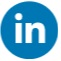# Data Types in Java1 likeData Types in Java

Data types specify the different sizes and values that can be stored in the variable. There are two types of data types in Java: primitive and non-primitive.

Primitive data types: The primitive data types include boolean, char, byte, short, int, long, float, and double.

Non-primitive data types: The non-primitive data types include Classes, Interfaces, and Arrays.

Java Primitive Data Type - In Java language, primitive data types are the building blocks of data manipulation. These are the most basic data types available in Java language.

There are 8 types of primitive data types:

Boolean data type
byte data type
char data type
short data type
int data type
long data type
float data type
double data type

Data Type Default size
boolean 1 bit
char 2 byte
byte 1 byte
short 2 byte
int         4 byte
long 8 byte
float 4 byte
double 8 byte

Boolean Data Type
The Boolean data type is used to store only two possible values: true and false. This data type is used for simple flags that track true/false conditions.

The Boolean data type specifies one bit of information, but its "size" can't be defined precisely.

Example:

Boolean one = false

Byte Data Type
The byte data type is an example of a primitive data type. It is an 8-bit signed two's complement integer. Its value range lies between -128 to 127 (inclusive). Its minimum value is -128 and the maximum value is 127. Its default value is 0.

The byte data type is used to save memory in large arrays where memory savings is most required. It saves space because a byte is 4 times smaller than an integer. It can also be used in place of the "int" data type.

Example:

byte a = 10, byte b = -20

Short Data Type
The short data type is a 16-bit signed two's complement integer. Its value range lies between -32,768 to 32,767 (inclusive). Its minimum value is -32,768 and its maximum value is 32,767. Its default value is 0.

The short data type can also be used to save memory just like the byte data type. A short data type is 2 times smaller than an integer.

Example:

short s = 10000, short r = -5000

Int Data Type
The int data type is a 32-bit signed two's complement integer. Its value range lies between - 2,147,483,648 (-2^31) to 2,147,483,647 (2^31 -1) (inclusive). Its minimum value is - 2,147,483,648and maximum value is 2,147,483,647. Its default value is 0.

The int data type is generally used as a default data type for integral values unless there is no problem with memory.

Example:
int a = 100000, int b = -200000

Long Data Type
The long data type is a 64-bit two's complement integer. Its value-range lies between -9,223,372,036,854,775,808(-2^63) to 9,223,372,036,854,775,807(2^63 -1)(inclusive). Its minimum value is - 9,223,372,036,854,775,808and maximum value is 9,223,372,036,854,775,807. Its default value is 0. The long data type is used when you need a range of values more than those provided by int.

Example:
long a = 100000L, long b = -200000L

Float Data Type
The float data type is a single-precision 32-bit IEEE 754 floating point. Its value range is unlimited. It is recommended to use a float (instead of a double) if you need to save memory in large arrays of floating point numbers. The float data type should never be used for precise values, such as currency. Its default value is 0.0F.

Example:
float f1 = 234.5f

Double Data Type
The double data type is a double-precision 64-bit IEEE 754 floating point. Its value range is unlimited. The double data type is generally used for decimal values just like float. The double data type also should never be used for precise values, such as currency. Its default value is 0.0d.

Example:
double d1 = 12.3

Char Data Type
The char data type is a single 16-bit Unicode character. Its value range lies between '\u0000' (or 0) to '\uffff' (or 65,535 inclusive). The char data type is used to store characters.

Example:
char letterA = 'A'#### Recent lost & found:Create and manage your profileRefer an author and get bonus Learn morePublish any lost and found belongingsConnect with the authors & add your review commentsJoin us for Free to advertise for your business or Contact-us for more detailsJoin us for Free to publish your own blogs, articles or tutorials and get your Benefits